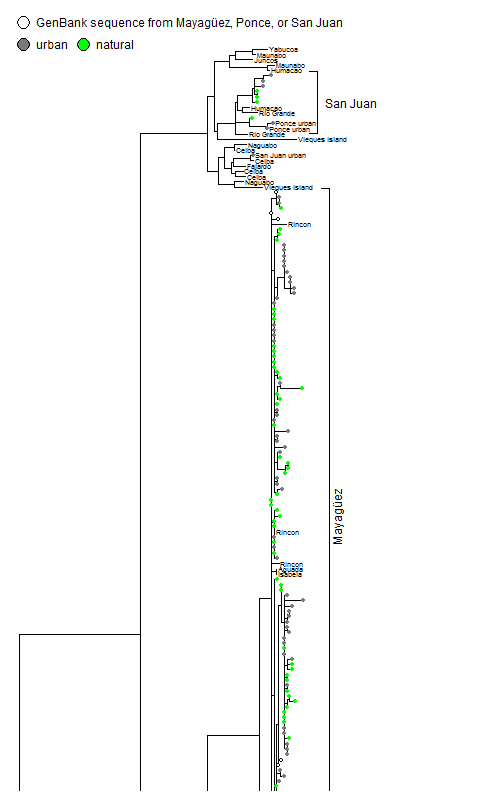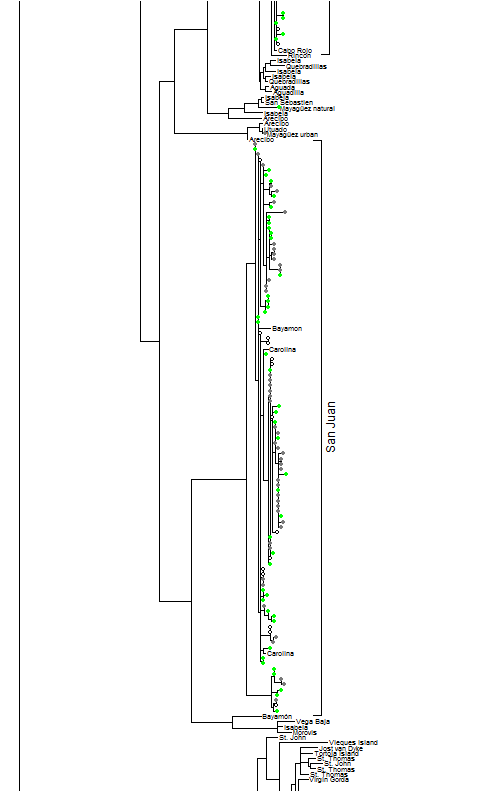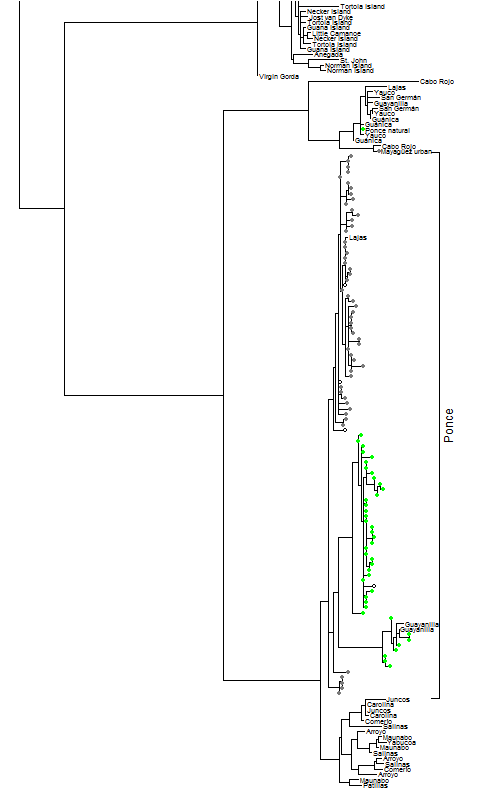## Tuesday, August 11, 2015

### Update to plotSimmap, fix to cladelabels, & plotting a tree across multiple pages

I have been working on producing a tree for the appendix of a manuscript in review at Evolution by my Ph.D. student Kristin Winchell. The tree is fairly big (433 tips), & yet it is necessary for the tip labels & symbols to be fairly legible in print so as not to obscure interpretation.

The demo below requires new versions of `plotSimmap`, as well as the function `split.plotTree` (not to be confused with `splitplotTree`), not presently in phytools, but described before on this blog. (Code here.)

``````foo<-function(){
par(fg="gray48")
nodelabels(node=uu,pie=matrix(rep(1,length(uu)),length(uu),1),
piecol="gray48",cex=0.2)
par(fg="green")
nodelabels(node=nn,pie=matrix(rep(1,length(uu)),length(uu),1),
piecol="green",cex=0.2)
par(fg="black")
nodelabels(node=mm,pie=matrix(rep(1,length(mm)),length(mm),1),
piecol="white",cex=0.2)
orientation="horizontal")
prompt=FALSE,x=0,y=438,shape="circle")
prompt=FALSE,x=0.15*max(nodeHeights(tree)),y=438,
shape="circle")
"GenBank sequence from MayagÃ¼ez, Ponce, or San Juan"),
prompt=FALSE,x=0,y=442,shape="circle")
}

tip.spacing<-c(1:146,149:295,298:437)
split.plotTree(tree,fsize=0.6,lwd=1,xlim=c(0,0.25),
split=c(0.33,0.668),fn=foo,tips=tip.spacing,y.lim=c(0,445))
``````The key updates to `cladelabels` and `plotSimmap` were the following:

1) In `plotSimmap` I made the small change to permit the vector giving the desired terminal edge vertical position (`tips`) to not have names, in which case it would be assumed to have the order given by the `1:Ntip(tree)` node indices. This is because in its current iteration it fails if some tip labels are repeating (as they are in this tree).

2) In `cladelabels` the function (erroneously) assumed by default that the tips have y positions `1:Ntip(tree)`.

The purpose of the two updates was to allow the multiple for greater spacing of tip labels across the splits between pages to ensure that the edges, tip labels, or tip symbols plotted cleanly.

A higher quality (PDF) version can be seen:

``````pdf(file="cristatellus-split.plotTree.pdf",height=11,width=7)
split.plotTree(tree,fsize=0.6,lwd=1,xlim=c(0,0.25),
split=c(0.33,0.668),fn=foo,tips=tip.spacing,y.lim=c(0,445))
dev.off()
``````
``````## png
##   2
``````

here.